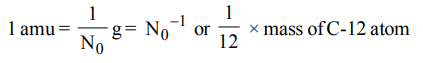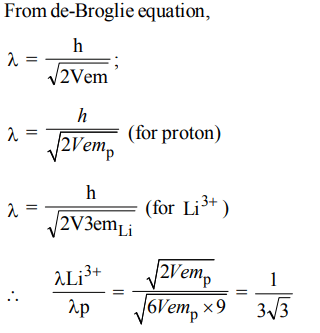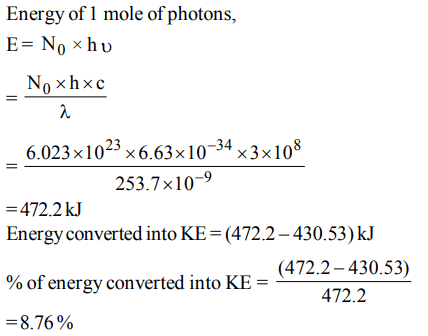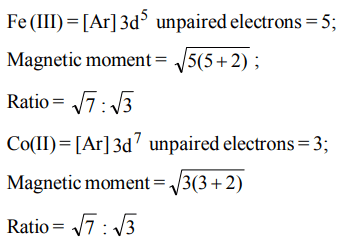## Atomic Structure Questions and Answers Part-20

1. A body of mass 10 mg is moving with a velocity of $100 ms^{-1}$ . The wavelength of de-Broglie's wave associated with it would be
a) $6.63 ×10^{-35}m$
b) $6.63 ×10^{-31}m$
c) $6.63 ×10^{-37}m$
d) $6.63 ×10^{-34}m$

Explanation:2. If $N_{0}$ represents the Avogadro number, then which of the following represent correct value of one atomic mass unit.
a) $N_{0}*10^{-3} kg$
b) $N_{0}g$
c) $N_0^{-1}g$
d) $\frac{1}{16}$ Mass of 0-16 atom

Explanation:3. If the shortest wavelength of the spectral line of H-atom in the Lyman series is X, then the longest wavelength of the line in Balmer series of $Li^{2+}$ is
a) 9x
b) $\frac{x}{9}$
c) $\frac{5x}{4}$
d) $\frac{4x}{5}$

Explanation:4. If the subsidiary quantum number of a sub-energy level is 4, the maximum and minimum values of the spin multiplicities are
a) 10, 2
b) 4, – 4
c) 10, 1
d) 9, 1

Explanation:5. $Li^{3+}$ and a proton are accelerated by the same potential, then de-Broglie wavelengths $\lambda_{Li}and \lambda_{p}$ have the ratio assume $\left(m_{Li}=9m_{p}\right)$
a) $1 :3\sqrt{3}$
b) 1 : 1
c) 1 : 2
d) 1 : 4

Explanation:6. One Bohr magnet on is equal to
a) $\frac{c}{4\pi hm_{e}}$
b) $\frac{h}{4\pi em_{e}}$
c) $\frac{eh}{4\pi m_{e}}$
d) $\frac{hc}{4\pi m_{e}}$

Explanation:7. The dissociation energy of H2 is $430.53 KJ mol^{-1}$  . If hydrogen is dissociated by illumination with radiation of wavelength 253.7 nm the fraction of the radiant energy which will be converted into kinetic energy is given by
a) 100%
b) 8.76%
c) 2.22%
d) 1.22%

Explanation:8. The magnetic moment of $M^{x+}$(atomic number M = 25) is $\sqrt{15}BM$. The number of unpaired elections and the value of x respectively are
a) 5, 2
b) 3, 2
c) 3, 4
d) 4, 3

Explanation:9. The threshold frequency of a metal is $1 ×10^{15}s^{-1}$ . The ratio of the maximum kinetic energies of the photoelectrons when the metal is irradiated with radiations of frequencies $1.5 ×10^{15}s^{-1}, 2.0 ×10^{15}s^{-1}$     respectively would be
a) 4 : 3
b) 1 : 2
c) 2 : 1
d) 3 : 4c) $\sqrt{7}: \sqrt{3}$
d) $\sqrt{3}: \sqrt{7}$